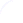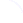Search
 Submission Procedure share: |Follow usavailable in:   PDF (211 kB) PS (384 kB)

get:
 Similar Docs BibTeX Write a comment
get:
 Links into Future

DOI:   10.3217/jucs-013-11-1655

### Satisfying Assignments of Random Boolean Constraint Satisfaction Problems: Clusters and Overlaps

Gabriel Istrate (eAustria Research Institute, Romania)

Abstract: The distribution of overlaps of solutions of a random constraint satisfaction problem (CSP) is an indicator of the overall geometry of its solution space. For random k-SAT, nonrigorous methods from Statistical Physics support the validity of the one step replica symmetry breaking approach. Some of these predictions were rigorously confirmed in [Mézard et al. 2005a] [Mézard et al. 2005b]. There it is proved that the overlap distribution of random k-SAT, k ≥ 9, has discontinuous support. Furthermore, Achlioptas and Ricci-Tersenghi [Achlioptas and Ricci-Tersenghi 2006] proved that, for random k-SAT, k ≥ 8, and constraint densities close enough to the phase transition:

- there exists an exponential number of clusters of satisfying assignments.

- the distance between satisfying assignments in different clusters is linear.

We aim to understand the structural properties of random CSP that lead to solution clustering. To this end, we prove two results on the cluster structure of solutions for binary CSP under the random model from [Molloy 2002]:

1. For all constraint sets S (described in [Creignou and Daudé 2004, Istrate 2005]) such that SAT (S) has a sharp threshold and all q ∈ (0, 1], q-overlap-SAT (S) has a sharp threshold. In other words the first step of the approach in [Mézard et al. 2005a] works in all nontrivial cases.

2. For any constraint density value c < 1, the set of solutions of a random instance of 2-SAT form with high probability a single cluster. Also, for and any q ∈ (0, 1] such an instance has with high probability two satisfying assignment of overlap ~ q. Thus, as expected from Statistical Physics predictions, the second step of the approach in [Mézard et al. 2005a] fails for 2-SAT.

Keywords: overlaps, random constraint satisfaction, sharp thresholds

Categories: G.2.1, G.3